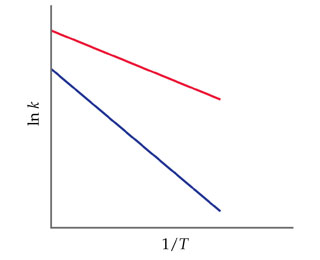# Problem: The accompanying graph shows plots of ln k versus 1/T for two different reactions. The plots have been extrapolated to the y-intercepts. Which reaction has the larger value for the frequency factor, A?

###### FREE Expert Solution

In the problem, we are asked to find which of the two plots has a larger value for the frequency factor (A).

The Arrhenius Equation shows the dependence of the rate constant on the pre-exponential factor, absolute temperature and activation energy of the reaction

Mathematically, the Arrhenius Equation is expressed as:

$\overline{){\mathbf{k}}{\mathbf{=}}{{\mathbf{Ae}}}^{\mathbf{-}{\mathbf{E}}_{\mathbf{a}}}{\mathbf{RT}}}}$

Where:
k is the rate constant
A is the frequency factor
Ea is the activation energy
R is the gas constant (8.314 J/mol•K )
T is the temperature

To solve this problem we must relate the Arrhenius Equation to the plot of the graph.

93% (416 ratings)###### Problem Details

The accompanying graph shows plots of ln k versus 1/T for two different reactions. The plots have been extrapolated to the y-intercepts.Which reaction has the larger value for the frequency factor, A?